## Jacobi Triple Product

The Jacobi triple product is the beautiful identity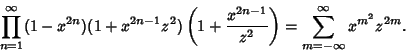(1)

In terms of the Q-Function, (1) is written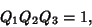(2)

which is one of the two Jacobi Identities. For the special case of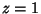, (1) becomes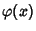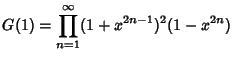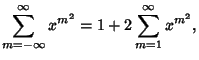(3)

whereis the one-variable Ramanujan Theta Function.

To prove the identity, define the function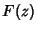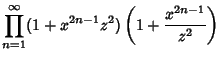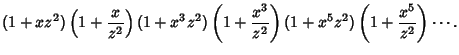(4)

Then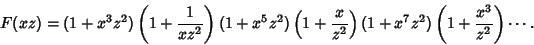(5)

Taking (5)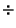(4),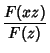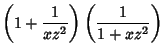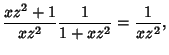(6)

which yields the fundamental relation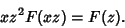(7)

Now define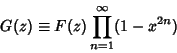(8)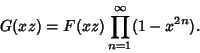(9)

Using (7), (9) becomes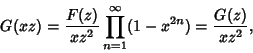(10)

so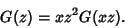(11)

Expand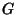in a Laurent Series. Sinceis an Even Function, the Laurent Series contains only even terms.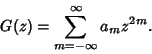(12)

Equation (11) then requires that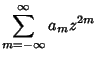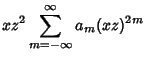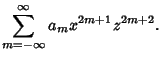(13)

This can be re-indexed with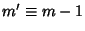on the left side of (13)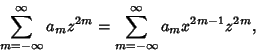(14)

which provides a Recurrence Relation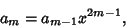(15)

so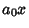(16)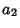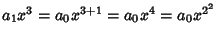(17)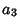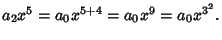(18)

The exponent grows greater by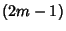for each increase inof 1. It is given by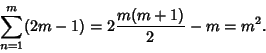(19)

Therefore,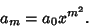(20)

This means that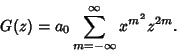(21)

The Coefficient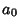must be determined by going back to (4) and (8) and letting. Then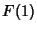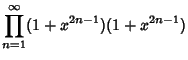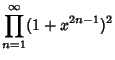(22)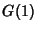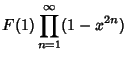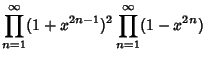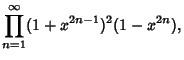(23)

since multiplication is Associative. It is clear from this expression that theterm must be 1, because all other terms will contain higher Powers of. Therefore,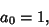(24)

so we have the Jacobi triple product,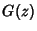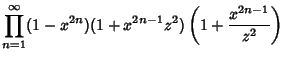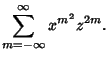(25)

See also Euler Identity, Jacobi Identities, Q-Function, Quintuple Product Identity, Ramanujan Psi Sum, Ramanujan Theta Functions, Schröter's Formula, Theta Function

References

Andrews, G. E.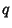-Series: Their Development and Application in Analysis, Number Theory, Combinatorics, Physics, and Computer Algebra. Providence, RI: Amer. Math. Soc., pp. 63-64, 1986.

Borwein, J. M. and Borwein, P. B. Jacobi's Triple Product and Some Number Theoretic Applications.'' Ch. 3 in Pi & the AGM: A Study in Analytic Number Theory and Computational Complexity. New York: Wiley, pp. 62-101, 1987.

Jacobi, C. G. J. Fundamentia Nova Theoriae Functionum Ellipticarum. Regiomonti, Sumtibus fratrum Borntraeger, p. 90, 1829.

Whittaker, E. T. and Watson, G. N. A Course in Modern Analysis, 4th ed. Cambridge, England: Cambridge University Press, p. 470, 1990.